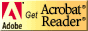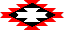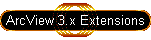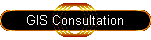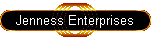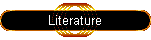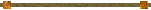#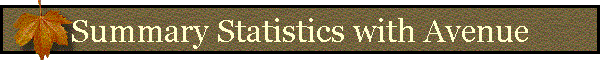#### Calculating Summary Statistics with Avenue

The Summary Statistics tool collects a series of True/False and Numerical parameters from the user and sends them to a script called “Jennessent.CalcFieldStats”, which does the necessary calculations and returns a list of results. The tool then prints those results up in a Report window for the user.

Avenue programmers can bypass the dialog and send values to the script directly if they wish, and then they will have the desired statistics directly available to them in a list. For example, many statistical calculations require such things as means, standard deviations, variances, quartiles, etc. The user may want to generate these values early in a script and then use them in later calculations. The “Jennessent.CalcFieldStats” script makes it simple to generate such values from data in a table.

This option is a little simpler than the standard Avenue method for generating statistics, which is to create a new file on the hard drive and then use the “Summarize” request to save statistics to that file. It also offers a larger variety of statistical output, including such things as Confidence Intervals, Standard Error of the Mean, Average Deviation, and Kurtosis/Skewness values. This option is also a little slower on large datasets, however, and it doesn’t divide up the dataset into subsets like “Summarize” does.

The function can be used with just a few lines of code: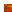ListOfResults = av.Run("Jennessent.CalcFieldStats", {ListOfInputParameters, theVTab, theField})

The object “theVTab” is a VTab object containing your data, and “theField” is a Field object in the VTab, reflecting the field you want to calculate statistics on.

The “ListOfInputParameters” must contain 20 values, most of which are Boolean (true/false) reflecting whether you want that particular statistic calculated:ListOfInputParameters = {CalcMean, CalcSEMean, CalcConInt, Con_Level, CalcMinimum, Calc1stQuart, CalcMedian, Calc3rdQuart, CalcMaximum, CalcVariance, CalcStandDev, CalcAvgDev, CalcSkewness, CalcSkewFish, CalcKurtosis, CalcKurtFish, CalcCount, CalcNumNull, CalcSum, CalcRange}

Where:CalcMean:  Boolean, True if you want to calculate the mean.CalcSEMean:  Boolean, True if you want to calculate the standard error of the mean.CalcConInt:  Boolean, True if you want to calculate confidence intervals of the mean.Con_Level:  Number, 0 <= p <= 1, where p = probability = (1 - α )CalcMinimum:  Boolean, True if you want to calculate the minimum value.Calc1stQuart:  Boolean, True if you want to calculate the 1st quartile.CalcMedian:  Boolean, True if you want to calculate the median.Calc3rdQuart:  Boolean, True if you want to calculate the 3rd quartile.CalcMaximum:  Boolean, True if you want to calculate the maximum value.CalcVariance:  Boolean, True if you want to calculate the variance.CalcStandDev:  Boolean, True if you want to calculate the standard deviation.CalcAvgDev:  Boolean, True if you want to calculate the absolute average deviation.CalcSkewness:  Boolean, True if you want to calculate the standard skewness.CalcSkewFish:  Boolean, True if you want to calculate the Fisher’s G1 skewness.CalcKurtosis:  Boolean, True if you want to calculate the standard kurtosis.CalcKurtFish:  Boolean, True if you want to calculate the Fisher’s G2 kurtosis.CalcCount:  Boolean, True if you want to calculate the total number of rows of data.CalcNumNull:  Boolean, True if you want to calculate the number of null values.CalcSum:  Boolean, True if you want to calculate the sum.CalcRange:  Boolean, True if you want to calculate the Range.

When the script finishes, it will return a list of 18 values to you representing the various statistics you requested. If you did not request a particular statistic, then it will not be calculated and the return list will contain a “nil” object in it’s place.

Return list: {Mean, Standard Error of Mean, Lower Confidence Level, Upper Confidence Level, Minimum, 1st Quartile, Median, 3rd Quartile, Maximum, Variance, Standard Deviation, Skewness, Fisher’s GI Skewness, Kurtosis, Fisher’s G2 Kurtosis, Record Count, Number of Null Values, Sum, Range}

For example: If you had a table of population demographic data containing a field of Annual Income values, and you were interested in the mean annual income plus a 95% confidence interval around that mean, then you would set up the code as follows:

theDemographyVTab = YourTable.GetVTab

theField = theDemographyVTab.FindField("Income")

theInputParameters = {True, False, True, 0.95, False, False, False, False, False, False, False, False, False, False, False, False, False, False, False, False}

theReturnList = av.Run("Jennessent.CalcFieldStats", {theInputParameters, theDemographyVTab , theField})

theMeanIncome = theReturnList.Get(0)

theLowerConfidenceLimit = theReturnList.Get(2).Get(0)

theUpperConfidenceLimit = theReturnList.Get(2).Get(1)

All the objects in “theReturnList” will be “nil” objects except for the ones at indices 0 and 2.  The Mean will be at index 0, the Lower 95% Confidence Limit will be the first item in index 2, and the Upper 95% Confidence Limit will be the second item in index 2.

In general, all the possible statistics can be obtained with the following lines of code.  Simply copy and paste the appropriate lines into your script:

theMean = theReturnList.Get(0)

theSEMean = theReturnList.Get(1)

if (Calculating_Confidence_Intervals)

LowerCI = theReturnList.Get(2).Get(0)

UpperCI = theReturnList.Get(2).Get(1)

end

theMinimum = theReturnList.Get(3)

theQ1 = theReturnList.Get(4)

theMedian = theReturnList.Get(5)

theQ3 = theReturnList.Get(6)

theMaximum = theReturnList.Get(7)

theVar = theReturnList.Get(8)

theStdDev = theReturnList.Get(9)

theAvgDev = theReturnList.Get(10)

theSkew = theReturnList.Get(11)

theFisherSkew = theReturnList.Get(12)

theKurt = theReturnList.Get(13)

theFisherKurt = theReturnList.Get(14)

theCount = theReturnList.Get(15)

theNumberNull = theReturnList.Get(16)

theSum = theReturnList.Get(17)

theRange = theReturnList.Get(18)Back to Statistics/Distributions | Probability Calculators | References

Discussion of Distribution Functions:
Probability Density Functions | Cumulative Distribution Functions | Quantile Functions